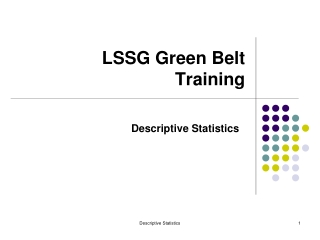DownloadDownload PresentationLSSG Green Belt Training

# LSSG Green Belt Training

Télécharger la présentation## LSSG Green Belt Training

- - - - - - - - - - - - - - - - - - - - - - - - - - - E N D - - - - - - - - - - - - - - - - - - - - - - - - - - -
##### Presentation Transcript

1. LSSG Green Belt Training Descriptive Statistics Descriptive Statistics

2. Describing Data: Summary Measures Measures of Central Location Mean, Median, Mode Measures of Variation Range, Variance and Standard Deviation Descriptive Statistics

3. Mean • It is the Arithmetic Average of data values: • The Most Common Measure of Central Tendency • Affected by Extreme Values (Outliers) + + · · · + x x x n = x å x = i 2 n i = Sample Mean i 1 n n 0 1 2 3 4 5 6 7 8 9 10 0 1 2 3 4 5 6 7 8 9 10 12 14 Mean = 5 Mean = 6 Descriptive Statistics

4. Median Median = 5 Median = 5 • Important Measure of Central Tendency • In an ordered array, the median is the “middle” number. • If n is odd, the median is the middle number. • If n is even, the median is the average of the 2 middle numbers. • Not Affected by Extreme Values 0 1 2 3 4 5 6 7 8 9 10 0 1 2 3 4 5 6 7 8 9 10 12 14 Descriptive Statistics

5. Mode No Mode Mode = 5 • A Measure of Central Tendency • Value that Occurs Most Often • Not Affected by Extreme Values • There May Not be a Mode • There May be Several Modes • Used for Either Numerical or Categorical Data 0 1 2 3 4 5 6 Descriptive Statistics

6. Measures Of Variability Range and Inter Quartile Range Variance and Standard Deviation Coefficient of Variation Descriptive Statistics

7. Range = 12 - 7 = 5 Range = 12 - 7 = 5 7 8 9 10 11 12 7 8 9 10 11 12 Range • Measure of Variation • Difference Between Largest & Smallest • Observations: • Range = Highest Value – Lowest Value • Ignores How Data Are Distributed: Descriptive Statistics

8. Inter Quartile Range • Difference between the 75th percentile (3rd Quartile) and the 25th percentile (1st Quartile) • Eliminates Effects of Outliers • Captures how data are distributed around the median (2nd Quartile) Q2 Q3 Q1 IQR Descriptive Statistics

9. ( ) 2 - m å X s 2 = i N ( ) 2 - å X X = i S2 - n 1 Variance • Important Measure of Variation • Shows Variation About the Mean • For the Population: • For the Sample: ) 2 m å s = ( ) 2 - X 2 = i s - n 1 Descriptive Statistics

10. ( ) 2 - m å X s = i N ( ) 2 - å X X = i s - n 1 Standard Deviation • Most Important Measure of Variation • Shows Variation About the Mean • For the Population: • For the Sample: For the Population: use N in the denominator. For the Sample : use n - 1 in the denominator. Descriptive Statistics

11. ( ) 2 - å X X = i s - n 1 Sample Standard Deviation For the Sample : use n - 1 in the denominator. Data: 10 12 14 15 17 18 18 24 n = 8 Mean =16 Sample Standard Deviation= 4.24 Descriptive Statistics

12. Comparing Standard Deviations Data A Mean = 15.5 s = 3.3 11 12 13 14 15 16 17 18 19 20 21 Data B Mean = 15.5 s = .92 11 12 13 14 15 16 17 18 19 20 21 Data C Mean = 15.5 s = 4.57 11 12 13 14 15 16 17 18 19 20 21 Descriptive Statistics

13. Coefficient of Variation • Relative Variation (adjusted for the mean) • Measured as a % • Adjusts for differences in magnitude of data • Comparison of variation across groups Descriptive Statistics

14. Comparing Coefficient of Variation Stock A: Average Price last year = \$50 Standard Deviation = \$5 Stock B: Average Price last year = \$100 Standard Deviation = \$5 Coefficient of Variation: Stock A: CV = 10% Stock B: CV = 5% Descriptive Statistics

15. Right-Skewed Left-Skewed Symmetric Mean Median Mode Mean = Median = Mode Mode Median Mean Shape of Distribution • Describes How Data Are Distributed • Measures of Shape: • Symmetric or skewed Descriptive Statistics

16. BOX PLOTS Captures Many Statistics in One Chart Mean Max Min Median Q1 Q3 Descriptive Statistics